# Using equations and inequalities to solve real life math problemsEquations and inequalities sometimes look boring; people do not really understand why they exist and why they are so extensively studied at school.

Equations help us solve many problems and some of the situations where equations must be used are really interesting.

In this blog post we will take up some fun problems which we will solve using equations and inequalities.

## Problem 1

You are buying a soccer ball in a store that costs 29 dollars (including taxes).

You are going to pay in cash and you only have townies and the cashier only has 5 dollar bills. So you can give 15 townies (30 dollars), but the cashier cannot give you a change of 1 dollar, because they only have 5 dollar bills.

What is the minimum number of townies you need to give to the cashier, so that the cashier could give you the correct change?

Solution:

So, let x be the number of townies and y be the number of 5 dollar bills.

The total amount of cash you’ll give is 2x (x is the number of townies)

The total amount of change you’ll receive is 5y (y is the number of 5 dollar bills)

Our equation then would look like: 2x – 5y=29

Let’s isolate for x: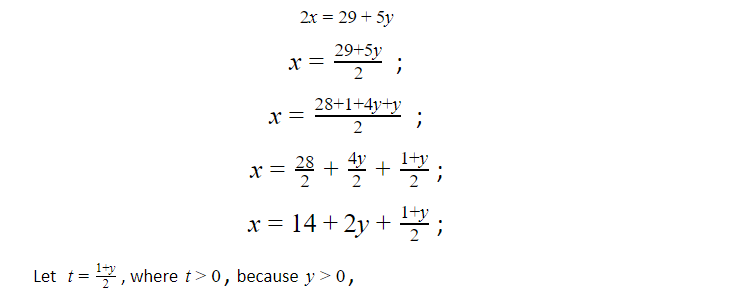t is a whole number (1,2,3,4,5,….) and t should be as small as possible, because we need to find the smallest values of y and x.

Let’s find y in terms of t: y=2t – 1

Now, let’s get back to our initial equation and substitute y with 2t – 1Now, let’s bring back our initial equation and substitute x with 12+5t as from the above statement.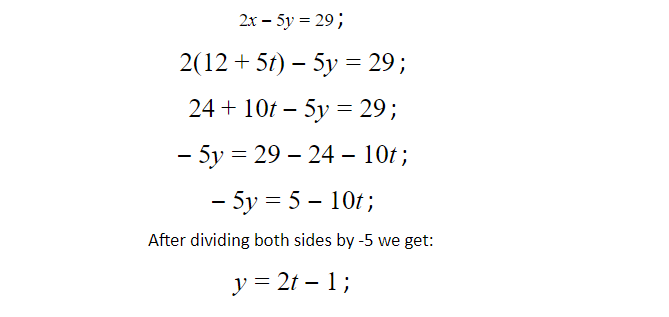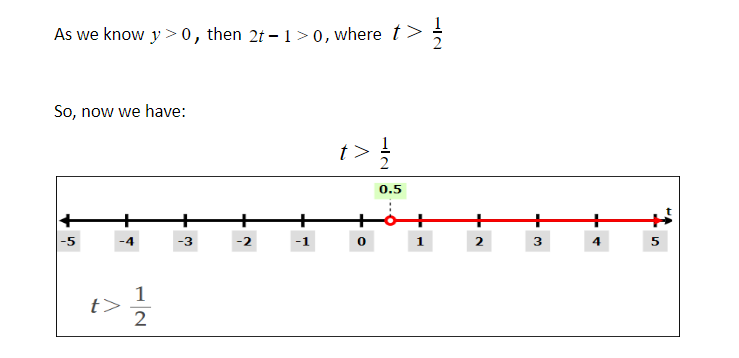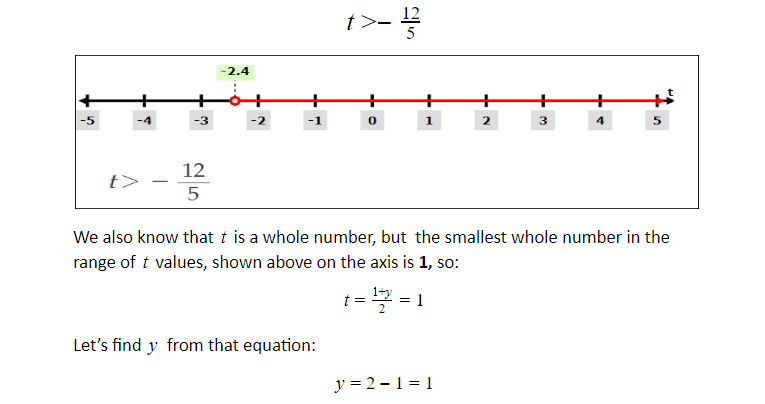Let’s find x using initial equation:So, you need to give 17 townies to the cashier and the cashier will give you a one 5 dollar bill.

Let’s check:

If you give 17 townies to the cashier, it will be 34 dollars, so the cashier will give you a change of a 5 dollar bill.

34 – 5=29

We have solved the first problem!

## Problem 2

Your mom is asking you to buy some fruits. She gave you 50 dollars to buy watermelons, apples and peaches – total of 100 fruits.

The prices are:

watermelons – 5 dollars/each, apples – 1 dollar/each and peaches – 10 cents/each.

How many apples, watermelons and peaches could you buy?

Solution:

Let

x represent the quantity of watermelons;

Y represent the quantity of apples;

Z represent the quantity of peaches.

Let’s create 2 equations: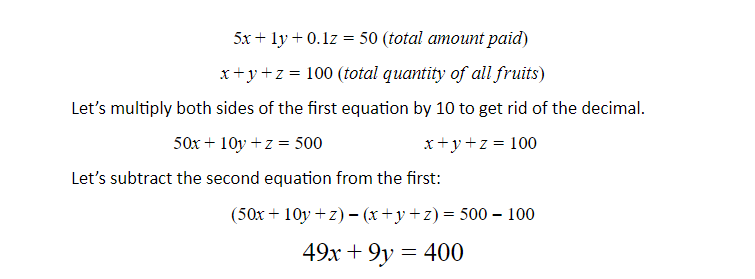Let’s now represent y:Let’s find y in terms of t: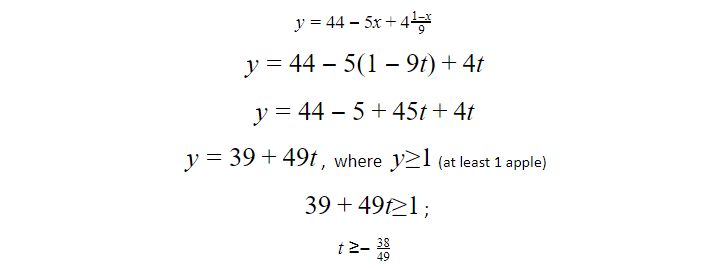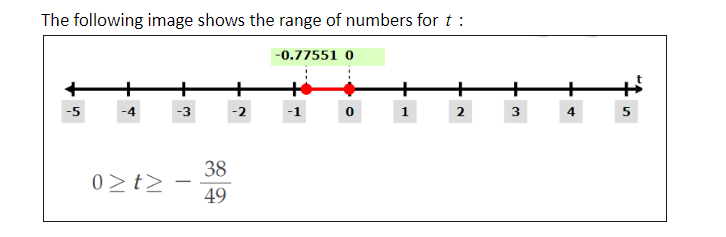Let’s get back to equation y=39 + 49t

As we know y ≥1 (at least 1 apple) and has to be a whole number, then 49t has to be an integer value (negative or positive or 0 number).

49t =7×7×t,

As we know 7 is a prime number(whose only factors are 1 and itself), so t needs to be an integer value as well, so that 49t can be an integer value and according to our above chart the only integer value for t is 0.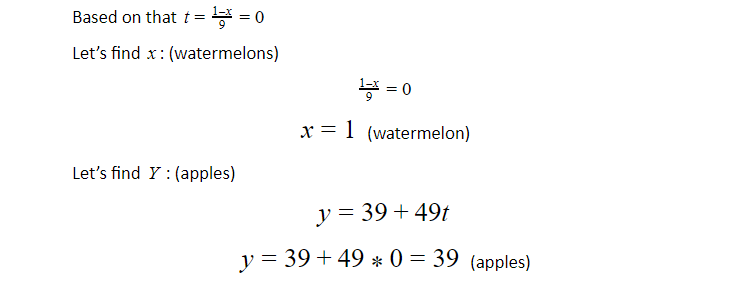So, you could purchase 1 watermelon, 39 apples and 60 peaches.

There is a huge amount of such interesting problems, especially in finances, construction and science that can be solved using equations and inequalities.

IntoMath Grade 8 and 9 mini-courses have lessons on solving linear equations and applications of linear equations – check them out!

0